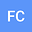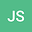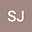Integral transforms of the Hilfer-type fractional derivatives
•••## Abstract

In this paper, some important properties concerning the Hilfer-type fractional derivatives are discussed. Integral transforms for these operators are derived as particular cases of the Jafari transform. These integral transforms are used to derive a fractional version of the fundamental theorem of calculus.

Keywords: Integral transforms, Jafari transform, $$\kappa$$-gamma function, $$\kappa$$-beta function, $$\kappa$$-Hilfer fractional derivative, $$\kappa$$-Riesz fractional derivative, $$\kappa$$-fractional operators, $$(\kappa,\rho)$$-Hilfer fractional derivative.